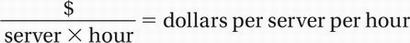# SAT Math Multiple Choice Question 648: Answer and Explanation

### Test Information

Question: 648

3. An information technology company estimates the cost of a project, in dollars, using the expression 240 1 3nt, where n is the number of computer servers working on the project and t is the total time, in hours, the project will take using n servers. Which of the following is the best interpretation of the number 3 in the expression?

• A. Each server costs the company \$3 per hour to run.
• B. A minimum of 3 servers will work on the project.
• C. The price of the project increases by \$3 every hour.
• D. Each server can work 3 hours per day.

Explanation:

A

Algebra (interpreting formulas) EASY-MEDIUM

One way to analyze a formula is by "dimensional analysis." This is simply a way to check that the units in the formula represent what they are supposed to represent. For instance, we are told that the expression 240 + 3nt represents the cost, in dollars, of the project. This means that both terms in this expression must be in dollars. Notice, also, that n is the number of servers and t is the number of hours. Let's use a ? to represent the units of the number 3 in the expression 3nt. Translating the term into units, this gives us

(?)(servers)(hours) = \$

Divide by (servers)(hours): _______________? =In other words, it costs the company 3 dollars per hour per server.